I.4.7

6/5/2021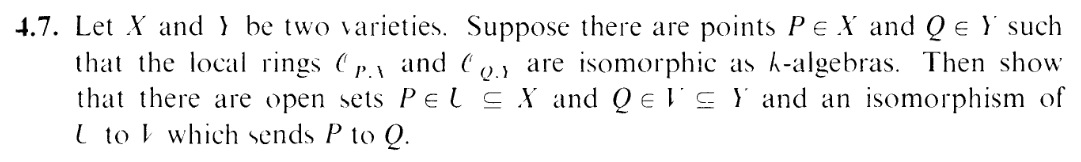IT'S MY LIIIIIIIIIIIIIIIFE AND IT'S NOW OR NEVERRRRRRRRRR. I AIN'T GONNA LIVE FOREVERRRRRR. I JUST WANNA LIVE WHILE I'M ALIIIIIIIIIIVE. GET OUT OF MY FACE. Listen: I've recovered from yesterday morning's CIA funded orgy of leafblowers. It was short lived. I went outside, was flanked by WEEEEEEEEEEEEEEEEEEEAAAAAAAAAAAAAAAAAAAAHHHHHHH at every corner, and even after it was over, I was so frustrated that it sabotaged the next few hours. However, by the afternoon, I recovered my default state. And now, on the next day, I'm thinking back on my silly "I NEED TO MOVE THE FUCK OUT OF HERE" sentiments. Had I simply stayed inside during that period of time, I'd have been fine. "I NEED TO MOVE THE FUCK OUT OF HERE" was just an impulsive, irrational reaction.

Hence, I'm moving cause of the leafblowers, and only the leafblowers. Wait, what? Yes. You can write "leafblowers" as the impetus on the official records. And suppose where I end up moving just has more leafblowers? Then I'll move again. Yes, that's it. Duh. It's simple! Listen: I'm not smart. All your complicated cost benefit/analysis on making a big decision makes my brain shut down. I can't think in terms of a spreadsheet. I'm not going to be able to do something if I actually have to think it through. All that results in is floundering in indecision like I've been for the past 5 years. I'm not smart enough to figure out the right/optimal/wise/moral thing. I'm stagnating and running outta time. If you're of my elk, you're prolly starting to see that it's time to make risky decisions and screw yourself and maybe even others over, lest you vanish. Listen sister, if you actually wanna move, for the love of god don't move for a good reason.The way I figured this exercise out was by "reverse engineering" it. First note that we can assume X,Y are affine without loss of generality. Let's suppose I had a morphism ϕ : X Y . Then the induced map would look like

 ψ :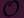P,Y →Q,X < U,f >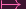< ϕ-1(U),f ∘ ϕ >

Now the key point to note is that we let f be the coodinate functions, we can recover ϕ coordinate by coordinate:

 x1 ∘ ϕ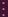xn ∘ ϕ

So this is what I'll do.

Let's suppose that we're given ψ, but not such ϕ.

 ψ :P,X →Q,Y

(yea I switched the domain and range from the initial example, sorry lol)

Let X An, Y Am. And let x1,,xn be coordinates for An and y1,,ym be coordinates for Am. Let's consider

 < V i,gi > = ψ(< X,xi >) (i = 1,…n) (1) < Ui,fi > = ψ-1(< Y,y i >) (i = 1,…m) (2)

Let U = Ui and V = V i, and then let

 ϕ : U → Y x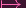(f1(x),…,fm(x))

(so that fi = yi ϕ, like it should be). Let's also try making an inverse:

 δ : V → X y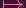(g1(y),…,gn(y))

Both are obviously morphisms (Lemma 3.6). Now let's show that, where they're defined, ϕ and δ are inverses:

For instance, given x = (X1,,Xn),

 δ ∘ ϕ(x) = δ(f1(x),…,fm(x)) (3) = (g1(f1(x),…,fm(x)),…,gn(f1(x),…,fm(x))) (4)

Now look at (1) and (2). And consider gi. Since it's a regular function, it can be written as (in some open set containing P; restrict V as needed) the fraction of polynomials l∕lover the yi. And thus

 gi =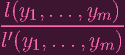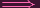ψ-1(g i) = ψ-1(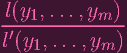)xi =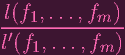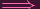Xi =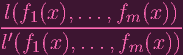(plugged in x to both sides) = gi(f1(x),…,fm(x))

Hence, (4) turns into just (X1,,Xn), which is just x, as needed. So δ ϕ(x) = x and an analogous argument shows that ϕ δ(y) = y.

Now I can't yet claim that ϕ and δ are isomorphic, as their domains and images don't necessarily match up. Which is why I said they're inverses where they're defined. Indeed what if ϕ(x) isn't contained in V ? Then δ(ϕ(x)) wouldn't even be defined. But I still need to keep U and V as open sets to satisfy the exercise. So I'll restrict the morphisms like this:

 ϕ′ : U ∩ ϕ-1(V ) → V δ′ : V ∩ δ-1(U) → U

Now consider x U ϕ-1(V ). Then ϕ(x) V . So we can apply δ on this element to get δ(ϕ(x)) = δ(ϕ(x)) = x U. So ϕ(x) δ-1(U). Which means that imϕ′⊂ V δ-1(U). Analogous reasoning shows that imδ′⊂ U ϕ-1(V ). So we can actually write the maps as

 ϕ′ : U ∩ ϕ-1(V ) → V ∩ δ-1(U) δ′ : V ∩ δ-1(U) → U ∩ ϕ-1(V )

Since δis a two-sided inverse for ϕ, ϕis an isomorphism. Phew.

Last thing: Does ϕ map P to Q? I got stuck here for a while, but I realized that in order to make statements specifically about P and Q, I should use the "locality" of the rings. So let mx and my be the unique maximal ideals ofP,X andP,Y . The uniqueness follows from the fact that they're local rings, and also note that they are given by all the elements that are 0 on P (respectively, Q). Now taking inspiration from example 1.4.4, note that the regular functions yi - Qi "determine" the point Q. Indeed they are 0 on Q, so yi - Qi my. Now where does ψ-1 send < Y,yi - Qi > to? By (2) and basic k algebra morphism properties, it gets send to < Ui,fi - Qi >. But since ψ-1 is an isomorphism, it maps maximal ideals to maximal ideals, therefore this element must be in mx. Which means that fi - Qi vanishes at P. I.e.

 fi(P) = Qi

Looking back at the definition of ϕ, it's now clear that ϕ maps P to Q.

PS: Koshka featured leafblowers in a past article, and actually has a rational argument against them that isn't just Bon Jovi lyrics.